# SMHS MethodsHeterogeneity MetaAnalysis

## Meta-analysis

### Overview

Meta-analysis is an approach to combine treatment effects across trials or studies into an aggregated treatment effect with higher statistical power than observed in each individual trials. It may detect HTE by testing for differences in treatment effects across similar RCTs. It requires that the individual treatment effects are similar to ensure pooling is meaningful. In the presence of large clinical or methodological differences between the trials, it may be to avoid meta-analyses. The presence of HTE across studies in a meta-analysis may be due to differences in the design or execution of the individual trials (e.g., randomization methods, patient selection criteria). Cochran's Q is a methods for detection of heterogeneity, which is computed as the weighted sum of squared differences between each study's treatment effect and the pooled effects across the studies. It is a barometer of inter-trial differences impacting the observed study result. A possible source of error in a meta-analysis is publication bias. Trial size may introduce publication bias since larger trials are more likely to be published. Language and accessibility represent other potential confounding factors. When the heterogeneity is not due to poor study design, it may be useful to optimize the treatment benefits for different cohorts of participants.

Cochran's Q statistics is the weighted sum of squares on a standardized scale8. The corresponding P value indicates the strength of the evidence of presence of heterogeneity. This test may have low power to detect heterogeneity sometimes and it is suggested to use a value of 0.10 as a cut-off for significance (Higgins et al., 2003). The Q statistics also may have too much power as a test of heterogeneity when the number of studies is large.

### Simulation Example 1

```# Install and Load library
install.packages("meta")
library(meta)

# Set number of studies
n.studies = 15

# number of treatments: case1, case2, control
n.trt = 3

# number of outcomes
n.event = 2

# simulate the (balanced) number of cases (case1 and case2) and controls in each study
ctl.group = rbinom(n = n.studies, size = 200, prob = 0.3)
case1.group = rbinom(n = n.studies, size = 200, prob = 0.3)
case2.group = rbinom(n = n.studies, size = 200, prob = 0.3)
```
```# Simulate the number of outcome events (e.g., deaths) and no events in the control group
event.ctl.group = rbinom(n = n.studies, size = ctl.group, prob = rep(0.1, length(ctl.group)))
noevent.ctl.group = ctl.group - event.ctl.group

# Simulate the number of events and no events in the case1 group
event.case1.group = rbinom(n = n.studies, size = case1.group, prob = rep(0.5, length(case1.group)))
noevent.case1.group = case1.group - event.case1.group
```
```# Simulate the number of events and no events in the case2 group
event.case2.group = rbinom(n = n.studies, size = case2.group, prob = rep(0.6, length(case2.group)))
noevent.case2.group = case2.group - event.case2.group
```
```# Run the univariate meta-analysis using metabin(), Meta-analysis of binary outcome data –
# Calculation of fixed and random effects estimates (risk ratio, odds ratio, risk difference or arcsine
# difference) for meta-analyses with binary outcome data.   Mantel-Haenszel (MH),
#  inverse variance and Peto method are available for pooling.
```
```# method = A character string indicating which method is to be used for pooling of studies.
# one of "MH" , "Inverse" , or "Cochran"
# sm = A character string indicating which summary measure (“OR”, "RR" "RD"=risk difference) is to be
# used for pooling of studies
```
```# Control vs. Case1, n.e and n.c are numbers in experimental and control groups
meta.ctr_case1 <- metabin(event.e = event.case1.group, n.e = case1.group, event.c = event.ctl.group,
n.c = ctl.group, method = "MH", sm = "OR")
# in this case we use Odds Ratio, of the odds of death in the experimental and control studies
forest(meta.ctr_case1)
```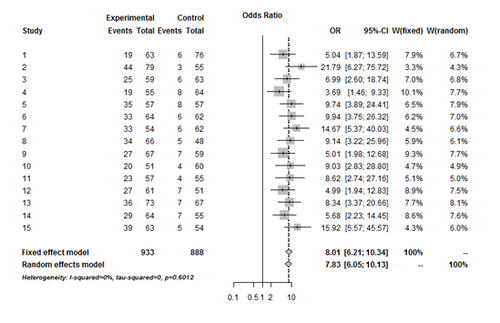```# Control vs. Case2
meta.ctr_case2 <- metabin(event.e = event.case2.group, n.e = case2.group, event.c = event.ctl.group,
n.c = ctl.group, method = "MH", sm = "OR")
forest(meta.ctr_case2)
```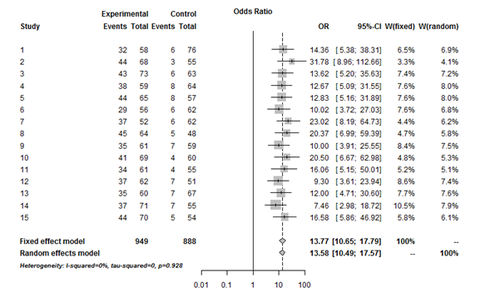```# Case1 vs. Case2
meta.case1_case2 <- metabin(event.e = event.case1.group, n.e = case1.group, event.c = event.case2.group,
n.c = case2.group, method = "MH", sm = "OR")
forest(meta.case1_case2)
summary(meta.case1_case2)
```
```Test of heterogeneity:
Q 	d.f.  	p-value
11.99   	14   	0.6071
```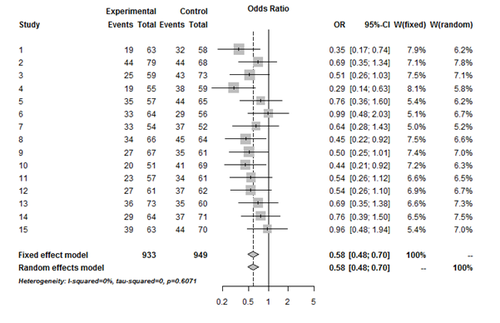The forest plot shows the I2 test indicates the evidence to reject the null hypothesis (no study heterogeneity and the fixed effects model should be used).

## Series of “N of 1” trials

This technique combines (a “series of”) n-of-1 trial data to identify HTE. An n-of-1 trial is a repeated crossover trial for a single patient, which randomly assigns the patient to one treatment vs. another for a given time period, after which the patient is re-randomized to treatment for the next time period, usually repeated for 4-6 time periods. Such trials are most feasibly done in chronic conditions, where little or no washout period is needed between treatments and treatment effects are identifiable in the short-term, such as pain or reliable surrogate markers. Combining data from identical n-of-1 trials across a set of patients enables the statistical analysis controlling for patient fixed or random effects, covariates, centers, or sequence effects, see Figure below. These combined trials are often analyzed within a Bayesian context using shrinkage estimators that combine individual and group mean treatment effects to create a “posterior” individual mean treatment effect estimate which is a form of inverse variance-weighted average of the individual and group effects. Such trials are typically more expensive than standard RCTs on a per-patient basis, however, they require much smaller sample sizes, often less than 100 patients (due to the efficient individual-as-own-control design), and create individual treatment effect estimates that are not possible in a non-crossover design . For the individual patient, the treatment effect can be re-estimated after each time period, and the trial stopped at any point when the more effective treatment is identified with reasonable statistical certainty.

#### Example

A study involving 8 participants collected data across 30 days, in which 15 treatment days and 15 control days are randomly assigned within each participant. The treatment effect is represented as a binary variable (control day=0; treatment day=1). The outcome variable represents the response to the intervention within each of the 8 participants. Study employed a fixed-effects modeling. By creating N − 1 dummy-coded variables representing the N=8 participants, where the last (i=8) participant serves as the reference (i.e., as the model intercept). So, each dummy-coded variable represents the difference between each participant (i) and the 8th participant. Thus, all other patients' values will be relative to the values of the 8th (reference) subject. The overall differences across participants in fixed effects can be evaluated with multiple degree-of-freedom F-tests.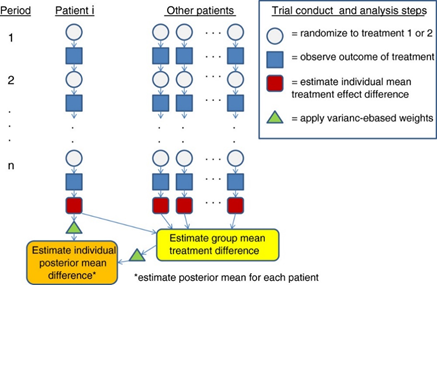ID Day Tx SelfEff SelfEff25 WPSS SocSuppt PMss PMss3 PhyAct 1 1 1 33 8 0.97 5.00 4.03 1.03 53 1 2 1 33 8 -0.17 3.87 4.03 1.03 73 1 3 0 33 8 0.81 4.84 4.03 1.03 23 1 4 0 33 8 -0.41 3.62 4.03 1.03 36 ... ... ... ... ... ... ... ... ... ...

Complete data is available in the Appendix.

Data Summary
 Intercept Constant Physical Activity PhyAct Intervention Tx WP Social Support WPSS PM Social Support (1-3) PMss3 Self Efficacy SelfEff25
```rm(list=ls())
attach(Nof1)
```
 ID Day Tx SelfEff SelfEff25 WPSS SocSuppt PMss PMss3 PhyAct 1 1 1 1 33 8 0.97 5.00 4.03 1.03 53 2 1 2 1 33 8 -0.17 3.87 4.03 1.03 73 3 1 3 0 33 8 0.81 4.84 4.03 1.03 23 4 1 4 0 33 8 -0.41 3.62 4.03 1.03 36 5 1 5 1 33 8 0.59 4.62 4.03 1.03 21 6 1 6 1 33 8 -1.16 2.87 4.03 1.03 0
```df.1 = data.frame(PhyAct, Tx, WPSS, PMss3, SelfEff25)
```
```# library("lme4")
```
```lm.1 = model.lmer <- lmer(PhyAct ~ Tx + SelfEff + Tx*SelfEff + (1|Day) + (1|ID) , data= df.1)
summary(lm.1)
```
```Linear mixed model fit by REML ['lmerMod']
Formula: PhyAct ~ Tx + SelfEff + Tx * SelfEff + (1 | Day) + (1 | ID)
Data: df.1
```
```REML criterion at convergence: 8820
```
Scaled Residuals
 Min 1Q Median 3Q Max

Random Effects
 Groups Name Variance Std.Dev. Day (Intercept) 0.0 0.00 ID (Intercept) 601.5 24.53 Residual 969.0 31.13

Number of obs: 900, groups: Day, 30; ID, 30

Fixed Effects
 Estimate Std. Error t value (Intercept) 38.3772 14.4738 2.651 Tx 4.0283 6.3745 0.632 SelfEff 0.5818 0.5942 0.979 Tx:SelfEff 0.9702 0.2617 3.708

Correlation of Fixed Effects
 (Intr) Tx SlfEff Tx -0.220 SelfEff -0.946 0.208 Tx:SelfEff 0.208 -0.946 -0.220

```# Model:  PhyAct = Tx + WPSS + PMss3 + Tx*WPSS + Tx*PMss3 + SelfEff25 + Tx*SelfEff25 + ε
lm.2 = lm(PhyAct ~ Tx + WPSS + PMss3 + Tx*WPSS + Tx*PMss3 + SelfEff25 + Tx*SelfEff25, df.1)
summary(lm.2)
```
```Call:
lm(formula = PhyAct ~ Tx + WPSS + PMss3 + Tx * WPSS + Tx * PMss3 +
SelfEff25 + Tx * SelfEff25, data = df.1)
```
Residuals
 Min 1Q Median 3Q Max -102.39 -28.24 -1.47 25.16 122.41
Coefficients
 Estimate Std. Error t value t|)\$ (Intercept) 52.0067 1.8080 28.764 < 2e-16 *** Tx 27.7366 2.5569 10.848 < 2e-16 *** WPSS 1.9631 2.4272 0.809 0.418853 PMss3 13.5110 2.7853 4.851 1.45e-06 *** SelfEff25 0.6289 0.2205 2.852 0.004439 ** Tx:WPSS 9.9114 3.4320 2.888 0.003971 ** Tx:PMss3 8.8422 3.9390 2.245 0.025025 * Tx:SelfEff25 1.0460 0.3118 3.354 0.000829 ***

[Using SAS (StudyI_Analyses.sas, StudyIIab_Analyses.sas)]

Type 3 Tests of Fixed Effects
 Effect Num DF Den DF F Value \$Pr>F\$ Tx 1 224 67.46 <.0001 ID 7 224 25.95 <.0001 Tx*ID 7 224 2.92 0.0060

## Quantile Treatment Effect (QTE)

QTE employs quantile regression estimation (QRE) to examine the central tendency and statistical dispersion of the treatment effect in a population. These may not be revealed by the conventional mean estimation in RCTs. For instance, patients with different comorbidity scores may respond differently to a treatment. Quantile regression has the ability to reveal HTE according to the ranking of patients’ comorbidity scores or some other relevant covariate by which patients may be ranked. Therefore, in an attempt to inform patient-centered care, quantile regression provides more information on the distribution of the treatment effect than typical conditional mean treatment effect estimation. QTE characterizes the heterogeneous treatment effect on individuals and groups across various positions in the distributions of different outcomes of interest. This unique feature has given quantile regression analysis substantial attention and has been employed across a wide range of applications, particularly when evaluating the economic effects of welfare reform.

One caveat of applying QRE in clinical trials for examining HTE is that the QTE doesn’t demonstrate the treatment effect for a given patient. Instead, it focuses on the treatment effect among subjects within the qth quantile, such as those who are exactly at the top 10th percent in terms of blood pressure or a depression score for some covariate of interest, for example, comorbidity score. It is not uncommon for the qth quantiles to be two different sets of patients before and after the treatment. For this reason, we have to assume that these two groups of patients are homogeneous if they were in the same quantiles.

Income-Food Expenditure Example: Let’s examine the Engel data (N=235) on the relationship between food expenditure (foodexp) and household income (income). We can plot the data and then explore the superposition of the six fitted quantile regression lines.

```install.packages("quantreg")
library(quantreg)
data(engel)
attach(engel)
```
 Income Foodexp 1 420.1577 255.8394 2 541.4117 310.9587 3 901.1575 485.6800 4 639.0802 402.9974 5 750.8756 495.5608 6 945.7989 633.7978

summary(engel)
 Income Foodexp Min 377.1 242.3 1st Qu. 638.9 429.7 Median 884.0 582.5 Mean 982.5 624.2 3rd Qu. 1164.0 743.9 Max 4957.8 2032.7

Note: If Y be a real valued random variable with cumulative distribution function FY(y)=P(Y≤ y), then the τ-quantile of Y is given by

QY(τ)=FY-1(τ)=inf{ y:FY(y)≥τ}

where 0≤τ≤1.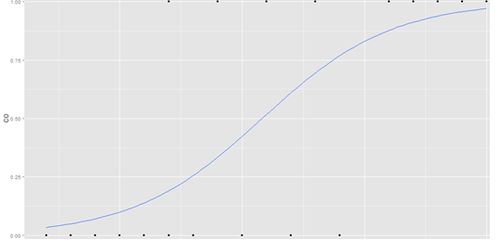```# (1) Graphics
plot(income, foodexp, cex=.25, type="n", xlab="Household Income", ylab="Food Expenditure")
points(income, foodexp, cex=.5, col="blue")
```
```# tau - the quantile(s) to be estimated, in the range from 0 to 1. An object "rq.process" and an object "rqs"
# are returned containing the matrix of coefficient estimates at the specified quantiles.
abline( rq(foodexp ~ income, tau=.5), col="blue")  	# Quantile Regression Model
```
```abline( lm(foodexp ~ income), lty=2, lwd=3, col="red") 	# linear model
taus <- c(0.05, 0.1, 0.25, 0.75, 0.90, 0.95)
colors <- rainbow(length(taus))
```
```models <- vector(mode = "list", length = length(taus)) # define a vector of models to store QR for diff taus
model.names <- vector(mode = "list", length = length(taus)) # define a vector model names
```
```for( i in 1:length(taus)){
modelsi <-  rq(foodexp ~ income, tau=taus[i])
var <- taus[i]
model.namesi <- paste("Model [", i , "]: tau=", var)
abline( modelsi, lwd=2, col= colorsi)
}
legend(3000, 1100, model.names, col= colors, pch= taus, bty='n', cex=.75)
```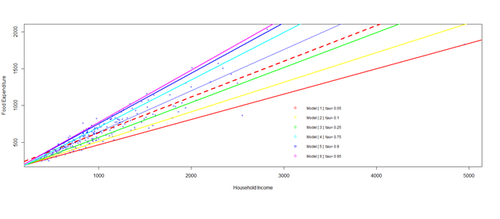```# (2) Inference about quantile regression coefficients.  As an alternative to the rank-inversion confidence intervals, we can obtain a table of coefficients, standard errors, t-statistics, and p-values using the summary function:
```
```summary(models3, se = "nid")
```
```Call: rq(formula = foodexp ~ income, tau = taus[i])
```
```tau:  0.25
```
Coefficients
 Value Std. Error t Value t|)\$ (Intercept) 95.48354 21.39237 4.46344 0.00001 Income 0.47410 0.02906 16.31729 0.00000
```# Alternatively, we can use summary.rq to compute bootstrapped standard errors.
summary.rq(models3, se = "nid")
```
```Call: rq(formula = foodexp ~ income, tau = taus[i])
tau:  0.25
```
Coefficients
 Value Std. Error t Value t|)\$ (Intercept) 95.48354 21.39237 4.46344 0.00001 Income 0.47410 0.02906 16.31729 0.00000

## Nonparametric Regression Methods

Nonparametric regression enables dealing with HTE in RCTs. Different nonparametric methods, such as kernel smoothing methods and series methods, can be used to generate test statistics for examining the presence of HTE. A kernel method is a weighting scheme based on a kernel function (e.g. uniform, Gaussian). When evaluating the treatment effect of a patient in RCTs, the kernel method assigns larger weights to those observations with similar covariates. This is done because it is assumed that patients with similar covariates provide more relevant data on predicted treatment response. Examining participants that have different backgrounds (e.g., demographic, clinical), kernel smoothing methods utilize information from highly divergent participants when estimating a particular subject’s treatment effect. Lower weights are assigned to very different subjects and the kernel methods require choosing a set of smoothing parameters to group patients according to their relative degree of similarities. A drawback is that the corresponding proposed test statistics may be sensitive to the chosen bandwidths, which inhibits the interpretation of the results. Series methods use approximating functions (splines or power series of the explanatory variables) to construct test statistics. Compared to kernel smoothing methods, series methods normally have the advantage of computational convenience; however, the precision of test statistics depends on the number of terms selected in the series.

Canadian Wage Data Example: Nonparametric regression extends the classical parametric regression (e.g., lm, lmer) involving one continuous dependent variable, y, and (1 or more) continuous explanatory variable(s), x. Let’s start with a popular parametric model of a wage equation that we can extend to a fully nonparametric regression model. First, we will compare and contrast the parametric and nonparametric approach towards univariate regression and then proceed to multivariate regression.

Let’s use the Canadian cross-section wage data (cps71) consisting of a random sample taken from the 1971 Canadian Census for male individuals having common education (High-School). N=205 observations, 2 variables, the logarithm of the individual’s wage (logwage) and their age (age). The classical wage equation model includes a quadratic term of age.

```# install.packages("np")
library("np")
data("cps71")
```
```# (1) Linear Model -> R2  = 0.2308
model.lin <- lm( logwage ~ age + I(age^2), data = cps71)
summary(model.lin)
```
```Call:
lm(formula = logwage ~ age + I(age^2), data = cps71)
```
```Residuals:
Min      1Q  Median      3Q     Max
-2.4041 -0.1711  0.0884  0.3182  1.3940
```

Coefficients
 Estimate Std. Error t Value t|)\$ (Intercept) 10.0419773 0.4559986 22.022 < 2e-16 *** Age 0.1731310 0.0238317 7.265 7.96e-12 *** I(age^2) -0.0019771 0.0002898 -6.822 1.02e-10 ***
```Signif. codes:  0 ‘***’ 0.001 ‘**’ 0.01 ‘*’ 0.05 ‘.’ 0.1 ‘ ’ 1
```
```Residual standard error: 0.5608 on 202 degrees of freedom
Multiple R-squared:  0.2308,	Adjusted R-squared:  0.2232
F-statistic:  30.3 on 2 and 202 DF,  p-value: 3.103e-12
```
```# (2) Next, we consider the local linear nonparametric method employing cross-validated
# bandwidth selection and estimation in one step. Start with computing the least-squares
#  cross-validated bandwidths for the local constant estimator (default).
# Note that R2 = 0.3108675
bandwidth <- npregbw(formula= logwage ~ age, data = cps71)
model.np <- npreg(bandwidth,  regtype = "ll",  bwmethod = "cv.aic", gradients = TRUE, data = cps71)
summary(model.np)
```
```Regression Data: 205 training points, in 1 variable(s) age
Bandwidth(s): 1.892157
Kernel Regression Estimator: Local-Constant
Bandwidth Type: Fixed
Residual standard error: 0.5307943
R-squared: 0.3108675
Continuous Kernel Type: Second-Order Gaussian
No. Continuous Explanatory Vars.: 1
```
```# NP model significance may be tested by
npsigtest(model.np)
```
```Kernel Regression Significance Test
Type I Test with IID Bootstrap (399 replications, Pivot=TRUE, joint=FALSE)
Explanatory variables tested for significance: age (1)
```
```              age
Bandwidth(s): 1.892157
```
```Individual Significance Tests
P Value:
age < 2.22e-16 ***
```
```# So, as was the case for the linear parametric model, Age is significant in the local linear NP-model
```
```# (3) Graphical comparison of parametric and nonparametric models.
plot(cps71\$\\$\$age, cps71\$\\$\$logwage, xlab = "age", ylab = "log(wage)", cex=.1)
lines(cps71\$\\$\$age, fitted(model.lin), lty = 2, col = " red")
lines(cps71\$\\$\$age, fitted(model.np), lty = 1, col = "blue")
legend("topright", c("Data", "Linear", "Non-linear"), col=c("Black", "Red", "Blue"), pch = c(1, 1, 1), bty='n', cex=.75)
```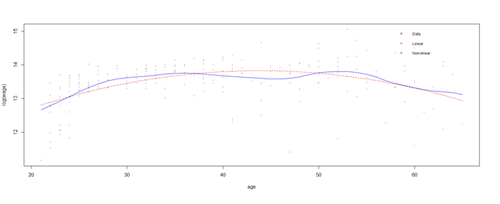```# some additional plots resenting the parametric (quadratic, dashed line) and the nonparametric estimates
# (solid line) of the regression function for the cps71 data.
plot(model.np, plot.errors.method = "asymptotic")
lines(cps71\$\\$\$age, coef(model.lin)+2*cps71\$\\$\$age*coef(model.lin), lty = 2, col = "red")
plot(model.np, gradients = TRUE, plot.errors.method = "asymptotic")
```
```# (4) using the Lin and NL models to generate predictions based on the obtained appropriate
# bandwidths and estimated a nonparametric model. We need to create a set of explanatory
# variables for which to generate predictions. These can be part of the original dataset or be
# outside its scope. Typically, we don’t have the outcome for the evaluation data and need only
# provide the explanatory variables for which predicted values are generated by the models.
# Occasionally, splitting the dataset into two independent samples (training/testing), allows estimation
# of a model on one sample, and evaluation of its performance on another.
```
```cps.eval.data <- data.frame(age = seq(10,70, by=10)) # simulate some explanatory X values (ages)
pred.lin <- predict(model.lin, newdata = cps.eval.data)		# Linear Prediction of log(Wage)
pred.np <- predict(model.np, newdata = cps.eval.data)		# non-Linear Prediction of log(Wage)
plot(pred.lin, pred.np)
abline(lm(pred.np ~ pred.lin))
```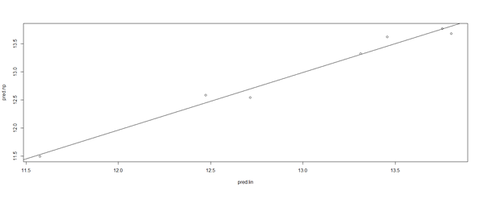. . .

## Predictive risk models

Predictive risk models represent a class of methods for identifying potential for HTE when the individual patient risk for disease-related events at baseline depends on observed factors. For instance, common measures are disease staging criteria, such as those used in COPD or heart failure, Framingham risk scores for cardiovascular event risk, or genetic variations, e.g., HER2 for breast cancer. Initial predictive risk modeling, aka risk function estimation, is often performed without accounting for treatment effects. Least squares or Cox proportional hazards regression methods are appropriate in many cases and provide relatively more interpretable risk functions, but rely on linearity assumptions and may not provide optimal predictive metrics. Partial least squares is an extension of least squares methods that can reduce the dimensionality of the predictor space by interposing latent variables, predicted by linear combinations of observable characteristics, as the intermediate predictors of one or more outcomes. Recursive partitioning, such as random forests, support vector machines, and neural networks represent latter methods with better predictive power than linear methods. Risk function estimation can range from highly exploratory analyses to near meta-analytic model validation, and may be useful at any stage of product development.

HIV Example: The “hmohiv” dataset represents a study of HIV positive patients examining whether there was a difference in survival times of HIV positive patients between a cohort using intravenous drugs (drug=1) and a cohort not using the IV drug (drug=0). The hmohiv data includes the following variables:

 ID Time Age Drug Censor Entdate Enddate 1 5 46 0 1 5/15/1990 10/14/1990 2 6 35 1 0 9/19/1989 3/20/1990 3 8 30 1 1 4/21/1991 12/20/1991 4 3 30 1 1 1/3/1991 4/4/1991 5 22 36 0 1 9/18/1989 7/19/1991 6 1 32 1 0 3/18/1991 4/17/1991 ... ... ... ... ... ... ...
```#cleaning up environment
rm(list=ls())
```
```# load survival library
library(survival)
```
```# load hmohiv data
attach(hmohiv)
```
```# Fit Cox proportional hazards regression model
cox.model <- coxph( Surv(time, censor) ~ drug, method="breslow")
fit.1 <- survfit(cox.model, newdata=drug.new)
```
```# construct a frame of the 2 cohorts IV_drug and no-IV-drug
drug.new<-data.frame(drug=c(0,1))
```
```# plot results
plot(fit.1, xlab="Survival Time (Months)", ylab="Survival Probability")
points(fit.1\$\\$\$time, fit.1\$\\$\$surv[,1], pch=1)
points(fit.1\$\\$\$time, fit.1\$\\$\$surv[,2], pch=2)
legend(40, .8, c("Drug Absent", "Drug Present"), pch=c(1,2))
```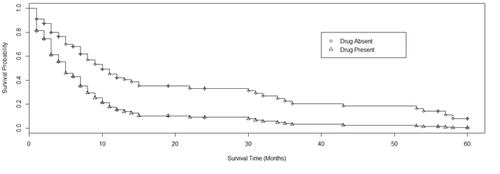```# to inslect the resulting Cox Proportional Hazard Model
cox.model
Call:
coxph(formula = Surv(time, censor) ~ drug, method = "breslow")
```
```     	    coef 	exp(coef) 	se(coef)   	z      	        p
drug 	0.779          2.18    		0.242 		3.22 	       0.0013
```
```Likelihood ratio test=10.2  on 1 df, p=0.00141  n= 100, number of events= 80
```